# Math in Focus Grade 3 Chapter 10 Practice 6 Answer Key Subtraction

Practice the problems of Math in Focus Grade 3 Workbook Answer Key Chapter 10 Practice 6 Subtraction to score better marks in the exam.

## Math in Focus Grade 3 Chapter 10 Practice 6 Answer Key Subtraction

Change dollars and cents to cents. Then subtract.

Example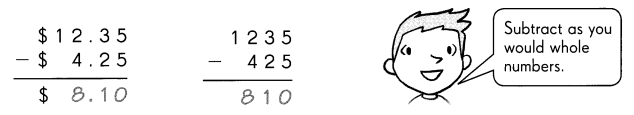Question 1.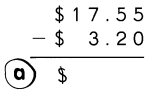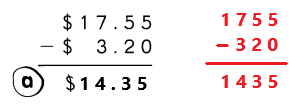Explanation:
First we have to change dollars and cents to cents. We know that 1 us dollar is equal to 100 us cents.
Convert $17.55 into 1755¢ and$3.20 into 320¢.
Subtract 320¢ from 1755¢ the difference is 1435¢.
The cents 1435¢ in dollar form as $14.35. Question 2.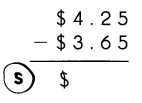Answer: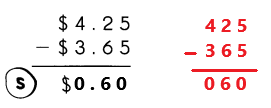Explanation: First we have to change dollars and cents to cents. We know that 1 us dollar is equal to 100 us cents. Convert$4.25 into 425¢ and $3.65 into 365¢. Subtract 365¢ from 425¢ the difference is 60¢. The cents 60¢ in dollar form as$0.60.

Question 3.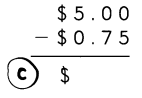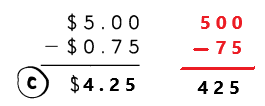Explanation:
First we have to change dollars and cents to cents. We know that 1 us dollar is equal to 100 us cents.
Convert $5.00 into 500¢ and$0.75 into 75¢.
Subtract 75¢ from 500¢ the difference is 425¢.
The cents 425¢ in dollar form as $4.25. Question 4.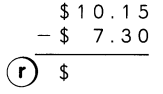Answer: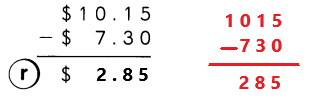Explanation: First we have to change dollars and cents to cents. We know that 1 us dollar is equal to 100 us cents. Convert$10.15 into 1015¢ and $7.30 into 730¢. Subtract 730¢ from 1015¢ the difference is 285¢. The cents 285¢ in dollar form as$2.85.

Question 5.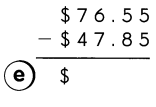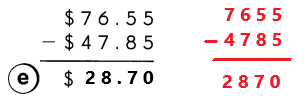Explanation:
First we have to change dollars and cents to cents. We know that 1 us dollar is equal to 100 us cents.
Convert $76.55 into 7655¢ and$47.85 into 4785¢.
Subtract 4785¢ from 7655¢ the difference is 2870¢.
The cents 2870¢ in dollar form as $28.70. Question 6.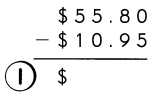Answer: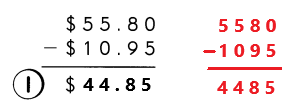Explanation: First we have to change dollars and cents to cents. We know that 1 us dollar is equal to 100 us cents. Convert$55.80 into 5580¢ and $10.95 into 1095¢. Subtract 1095¢ from 5580¢ the difference is 4485¢. The cents 4485¢ in dollar form as$44.85.

Question 7.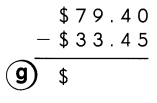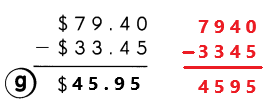Explanation:
First we have to change dollars and cents to cents. We know that 1 us dollar is equal to 100 us cents.
Convert $79.40 into 7940¢ and$33.45 into 3345¢.
Subtract 3345¢ from 7940¢ the difference is 4595¢.
The cents 4595¢ in dollar form as $45.95. Question 8.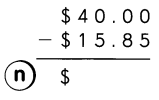Answer: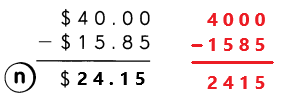Explanation: First we have to change dollars and cents to cents. We know that 1 us dollar is equal to 100 us cents. Convert$40.00 into 4000¢ and $15.85 into 1585¢. Subtract 1585¢ from 4000¢ the difference is 2415¢. The cents 2415¢ in dollar form as$24.15.

Write the letter that matches each answer to complete the sentence.

Question 9.
Let’s keep our national parks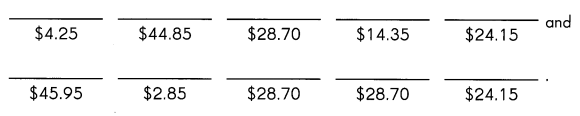Find the amount of change you will receive.

Question 10.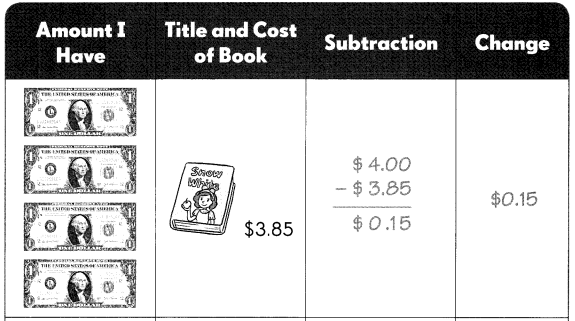I have four $1 notes. Multiply 4 with$1 the product is $4. The title of the book is snow white. The cost of the book is$3.85.
Subtract book cost from amount I have. Subtract $3.85 from$4 the difference is $0.15. I will receive the amount of change$0.15.

Question 11.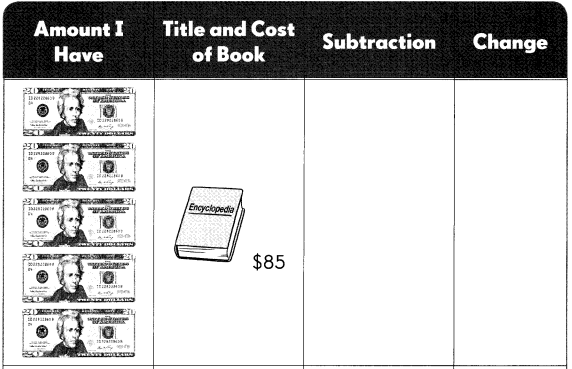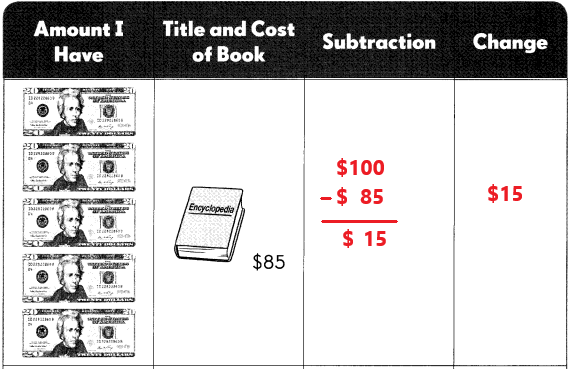Explanation:
I have five $20 notes. Multiply 5 with$20 the product is $100. The title of the book is Encyclopedia. The cost of the book is$85.
Subtract book cost from amount I have. Subtract $85 from$100 the difference is $15. I will receive the amount of change$15.

Question 12.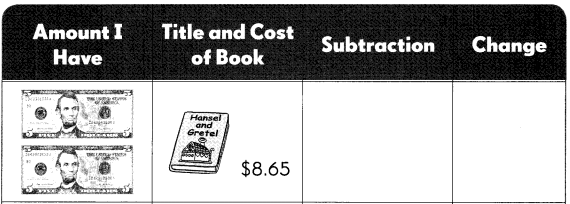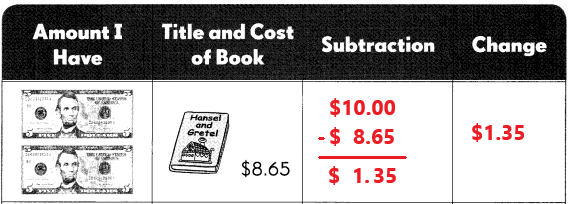I have two $5 notes. Multiply 2 with$5 the product is $10. The title of the book is Hansel and Gretel. The cost of the book is$8.65.
Subtract book cost from amount I have. Subtract $8.65 from$10 the difference is $1.35. I will receive the amount of change$1.35.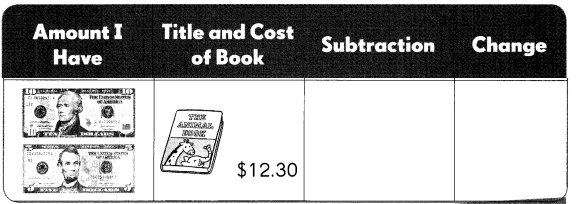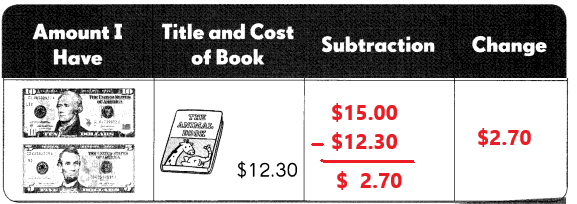I have one $10 note and one$5 note. Add $10 with$5 the sum is $15. The title of the book is The animal move. The cost of the book is$12.30.
Subtract book cost from amount I have. Subtract $12.30 from$15.00 the difference is $2.70. I will receive the amount of change$2.70.